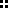### Functions in JavaScript

####Introduction

Though declaration and use of functions in JavaScript is simple and obeys rules common for other programming languages, a few details are worth recalling: function declaration, argument handling, scope of the variables and the function as data property.

####Declaring and calling a function

Compared to Java or C#, JavaScript does admit stand-alone functions. A function is created using the keyword function, a user-created function name and a list of comma-separated arguments in rounded brackets. The following example defines a function that returns the area of a rectangle based on the provided width w and height h:

``````
function area(w,h){
return w*h;
}
``````

JavaScript functions are dealt with the same way as in C or C++. For example, the line

``````
var s = area(2 ,3);
``````

assigns s the value 6. The return command is not required and is omitted if a function returns no value. The return value is then undefined. It is rather common to let the user call the functions dynamically from the page e. g. by including something like this:

``````
<a href="javascript:window.alert('The area of a 2 by 3
feet rectangle is '+area(2,3)+' feet!')">
Click here to learn something.
</a>
``````

####Arguments

A JavaScript function can be called with a different number of arguments then it is declared with, which can lead to unpleasant errors. This feature can also be well utilized, because the function arguments are stored in a field called arguments. An example looks like this:

``````
function multiply(){
var prod=1;
for (i=0; i<arguments.length; i++){
prod=prod*arguments[i];
}
return prod;
}
``````

The call multiply(1,2,3,4) returns 24, while multiply(1,2,3,4,"a") returns NaN.

####Scope

A variable introduced in a JavaScript function body is not limited to that function unless it is declared using var. This can cause problems, but can also be used for manipulation of the variable content by a function.

``````
var a=1;
function add(){
a=a+1;
return a;
}
``````

The function add() returns 2 after he first call but 3 after the second etc.

####Functions as data

A JavaScript function can be used as data, which is important for creating user-defined objects. The assignment

``````
var a=area
``````

Assigns the function area as a value to the variable a. Now, we can write a(2,3) instead of area(2,3), but we can also print the function definition using this simple trick:

``````
document.write(a + "");
``````

####References

David Barron: Scripting Languages. Wiley 2000

The ECMAScript scripting language. World Wide Web Consortium 1999